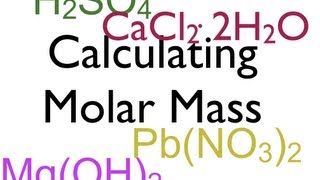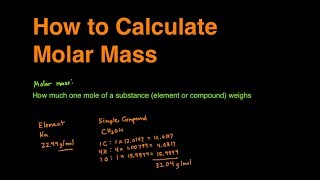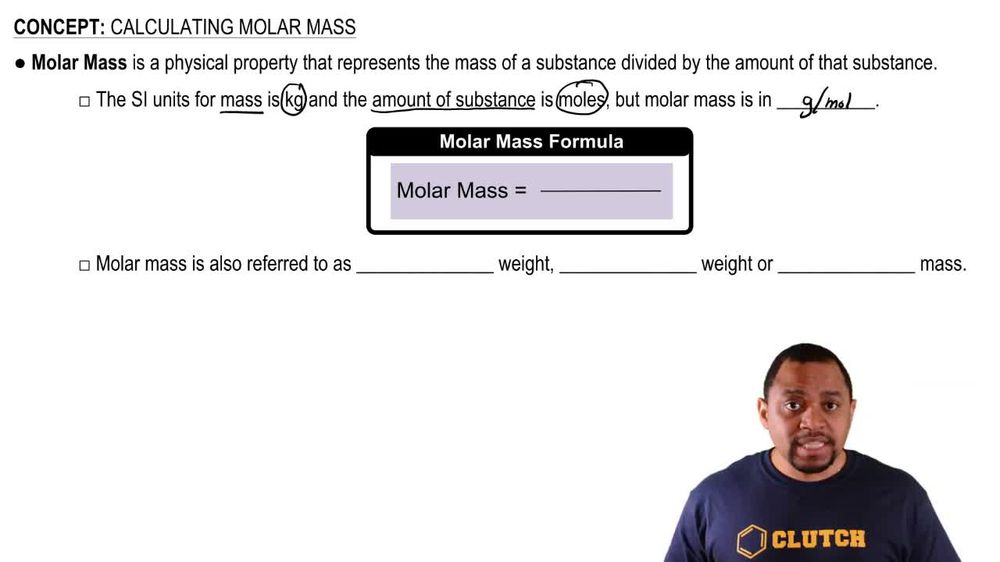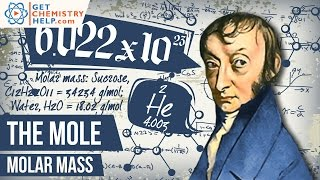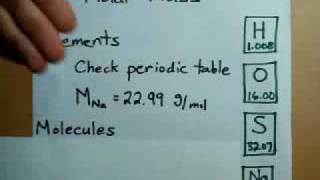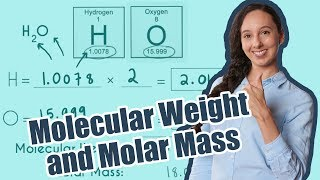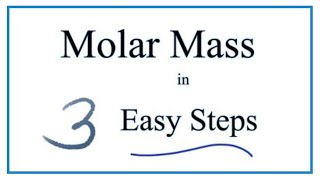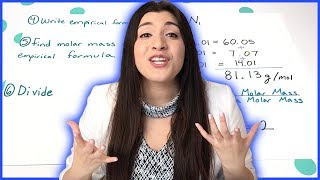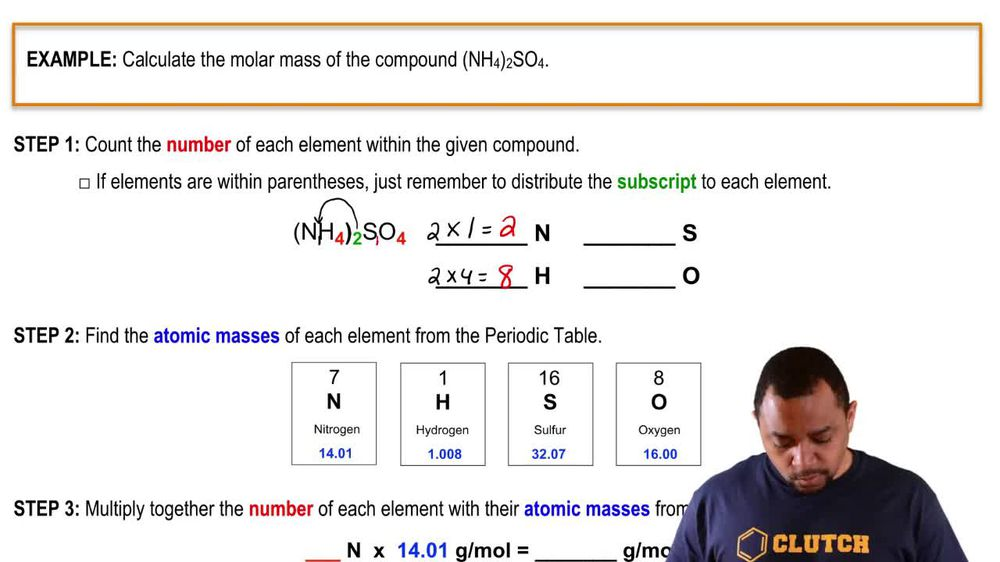Start typing, then use the up and down arrows to select an option from the list.
1. 2. Atoms & Elements2. Calculating Molar Mass
Problem

# If 6.02 * 1023 atoms of element Y have a mass of 83.80 g, what is the identity of Y?

Relevant Solution1m
Play a video:
Hey everyone, we're asked to identify element a. If 3.011 times 10 to the 23rd atoms of a have a mass of 59.36 g. To answer this question, we need to convert our atoms into moles and by converting our atoms into moles, we can divide our moles into our grams and determine the molar mass of element A. So solving this question, we're going to use our dimensional analysis, starting with 3.11 times 10 to the 23rd atoms. We're going to use avocados number in order to convert it into moles. Now we know that we have 6.22 times 10 to the 23rd atoms per one mole of our element A. Now, when we calculate this out and cancel out our unit, we end up with 0.5 mol. Now they told us that element a had a mass of 59.36 g. So solving for the molar mass of element A, we're going to take 59.36 g and divide that by 0. mol, This will get us to a molar mass of 118.7 g per mole. Now, when we look at our periodic table, we find that the element with the molar mass of 118.7 g per mole is going to be 10. Now, I hope this made sense And let us know if you have any questions